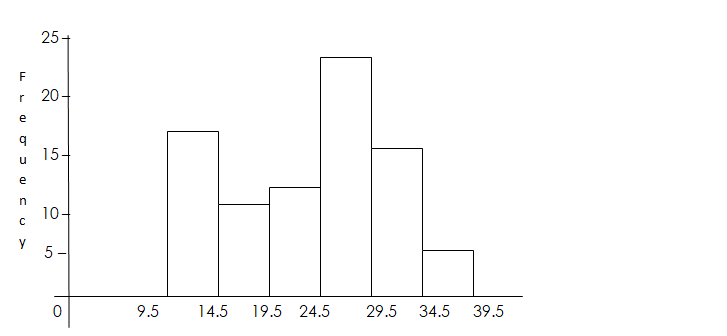# Statistics

Welcome to class!

In today’s class, we will be talking about statistics. Enjoy the class!

### StatisticsCONTENT

• Frequency Table.
• Cumulative Frequency Table.
• Histogram

When data has a large number of values, it is cumbersome to prepare its frequency table; hence the data are organized into classes or groups to overcome this problem. E.g. 0 – 4, 5 – 9, 10 – 14 e.g.

The range of the classes is first considered before we group the data. When data is divided into groups, it is called a grouped frequency distribution.

Grouped frequency distribution: The groups into which the data are arranged are called class intervals

E.g. 15 – 19

Class Limit: The number of each class intervals is called class limits of that interval.

Consider the class interval 20 – 24,

20 = lower class limit,    24 = upper class limit

Class Boundaries: When data is given to the nearest unit, the class interval 34 – 37, has a lower class boundary of 33.5 and upper-class boundary of 37.5.

Consider the intervals below: 20 – 24, 25 – 29 ETC. To obtain the class boundaries of 25 – 29,

24 + 25    = 24.5,     29 + 30 = 29.5

2                                2

Class Width: This is the difference between the upper-class boundary and the lower class boundary.

Class Marks: This is the centre or mid-point of any class interval. It is obtained by finding the average of the lower and upper limits. Find the class mark of the following class intervals 40 – 44, 45 – 49, 50 – 54 etc.

 Class Interval Class Mark 40 – 44 40 + 44 = 42 2 45 – 49 45 + 49 = 47 2
##### Cumulative Frequency Table

This is the table that shows the cumulative frequency of each of the classes and it is the running total of the frequencies class by class, giving the total frequency.

EXAMPLE: In a mock examination for the final year Chemistry class, the following were obtained by 50 students.

71   63   70   45   59   82   61   79   37   89

33   56   39   42   64   73   59   67   72   60

46   36   61   87   91   67   54   72   39   43

57   65   45   52   35   46   64   37   95   86

76   73   67   71   74   82   61   59   58   43

Using class interval 31 – 40, 41 – 50 … etc.   Construct a table showing the following columns: class interval, class boundary, class mark, frequency and cumulative frequency.

 Class interval Frequency Class boundary Class mark Cumulative Frequency 31 –  40 6 30.5 – 40.5 35.5 6 41 –  50 9 40.5 – 50.5 45.5 6 + 9     = 15 51 –  60 9 50.5 – 60.5 55.5 9 + 15   = 24 61 –  70 11 60.5 – 70.5 65.5 11 + 24 = 35 71 –  80 9 70.5 – 80.5 75.5 9 + 35   = 44 81 –  90 4 80.5 – 90.5 85.5 4 + 44   = 48 91 – 100 2 90.5 – 100.5 95.5 2 + 48   = 50

EVALUATION

The following figures show how many people visited an art gallery each day for 50 days.

Using class interval 11 – 20, 21 – 30 … etc.   Construct a table showing the following columns: class interval, boundary, class mark, frequency and cumulative frequency.

30   60   53   54   35   51   13   36   43   44

44   38   39   52   45   39   25   27   31   44

29   46   49   42   47   43   34   52   50   39

53   25   28   51   54   33   35   45   51   59

19   28   34   42   48   51   20   25   37   38

##### HISTOGRAM

This is a type of bar chart, each bar corresponding to one mark and with its length proportional to the frequency of that mark. The class marks or centres, class boundaries can be used on the variable scale. In histogram, the bars are joined together and must be of equal width, except when dealing with unequal class interval.

The following table shows the distribution of marks scored by a class of 80 students.

 Marks 10      –    14 15      –    19 20      –    24 25       –   29 30      –    34 35     –     39 Frequency 18 9 11 25 14 3

Draw a histogram for the distribution.

Solution

 Class Interval Class Mark Frequency 10        –      14 12 18 15        –      19 17 9 20        –      24 22 11 25        –      29 27 25 30        –      34 32 14 35        –      39 37 3##### Class boundaries

EVALUATION

Draw a histogram to illustrate the data shown below.

 Heights(cm) 120      – 129 130   –    139 140    –   149 150   –    159 160    –   169 170     –  179 Frequency 6 15 31 37 9 2

GENERAL EVALUATION

1. Construct a table showing the following columns: class interval, class boundary, class mark, frequency, and cumulative frequency for the distribution shown below.
 Shoe Sizes 5    –  9 10     –   14 15    –    19 20      –  24 25    – 29 30      –  34 35      –  39 No of students 5 7 6 2 3 4 3

In our next class, we will be talking more about Statistics.  We hope you enjoyed the class.

Should you have any further question, feel free to ask in the comment section below and trust us to respond as soon as possible.

How Can We Make ClassNotesNG Better - CLICK to Tell Us💃